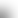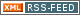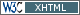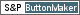www.LinuxHowtos.org howtos, tips&tricks and tutorials for linuxfrom small one page howto to huge articles all in one place

search text in:

Poll
Which linux distribution do you use?

poll results

Last additions:
using iotop to find disk usage hogs

using iotop to find disk usage hogs

words:

887

views:

149196

userrating:

average rating: 1.8 (88 votes) (1=very good 6=terrible)

May 25th. 2007:
Words

486

Views

245370

why adblockers are bad

Workaround and fixes for the current Core Dump Handling vulnerability affected kernels

Workaround and fixes for the current Core Dump Handling vulnerability affected kernels

words:

161

views:

128330

userrating:

average rating: 1.3 (28 votes) (1=very good 6=terrible)

April, 26th. 2006:

You are here: manpages

# EC_POINT_new

Section: OpenSSL (3)
Updated: 2017-05-25
Index Return to Main Contents

## NAME

EC_POINT_new, EC_POINT_free, EC_POINT_clear_free, EC_POINT_copy, EC_POINT_dup, EC_POINT_method_of, EC_POINT_set_to_infinity, EC_POINT_set_Jprojective_coordinates, EC_POINT_get_Jprojective_coordinates_GFp, EC_POINT_set_affine_coordinates_GFp, EC_POINT_get_affine_coordinates_GFp, EC_POINT_set_compressed_coordinates_GFp, EC_POINT_set_affine_coordinates_GF2m, EC_POINT_get_affine_coordinates_GF2m, EC_POINT_set_compressed_coordinates_GF2m, EC_POINT_point2oct, EC_POINT_oct2point, EC_POINT_point2bn, EC_POINT_bn2point, EC_POINT_point2hex, EC_POINT_hex2point - Functions for creating, destroying and manipulating EC_POINT objects.

## SYNOPSIS

``` #include <openssl/ec.h>
#include <openssl/bn.h>

EC_POINT *EC_POINT_new(const EC_GROUP *group);
void EC_POINT_free(EC_POINT *point);
void EC_POINT_clear_free(EC_POINT *point);
int EC_POINT_copy(EC_POINT *dst, const EC_POINT *src);
EC_POINT *EC_POINT_dup(const EC_POINT *src, const EC_GROUP *group);
const EC_METHOD *EC_POINT_method_of(const EC_POINT *point);
int EC_POINT_set_to_infinity(const EC_GROUP *group, EC_POINT *point);
int EC_POINT_set_Jprojective_coordinates_GFp(const EC_GROUP *group, EC_POINT *p,
const BIGNUM *x, const BIGNUM *y, const BIGNUM *z, BN_CTX *ctx);
int EC_POINT_get_Jprojective_coordinates_GFp(const EC_GROUP *group,
const EC_POINT *p, BIGNUM *x, BIGNUM *y, BIGNUM *z, BN_CTX *ctx);
int EC_POINT_set_affine_coordinates_GFp(const EC_GROUP *group, EC_POINT *p,
const BIGNUM *x, const BIGNUM *y, BN_CTX *ctx);
int EC_POINT_get_affine_coordinates_GFp(const EC_GROUP *group,
const EC_POINT *p, BIGNUM *x, BIGNUM *y, BN_CTX *ctx);
int EC_POINT_set_compressed_coordinates_GFp(const EC_GROUP *group, EC_POINT *p,
const BIGNUM *x, int y_bit, BN_CTX *ctx);
int EC_POINT_set_affine_coordinates_GF2m(const EC_GROUP *group, EC_POINT *p,
const BIGNUM *x, const BIGNUM *y, BN_CTX *ctx);
int EC_POINT_get_affine_coordinates_GF2m(const EC_GROUP *group,
const EC_POINT *p, BIGNUM *x, BIGNUM *y, BN_CTX *ctx);
int EC_POINT_set_compressed_coordinates_GF2m(const EC_GROUP *group, EC_POINT *p,
const BIGNUM *x, int y_bit, BN_CTX *ctx);
size_t EC_POINT_point2oct(const EC_GROUP *group, const EC_POINT *p,
point_conversion_form_t form,
unsigned char *buf, size_t len, BN_CTX *ctx);
int EC_POINT_oct2point(const EC_GROUP *group, EC_POINT *p,
const unsigned char *buf, size_t len, BN_CTX *ctx);
BIGNUM *EC_POINT_point2bn(const EC_GROUP *, const EC_POINT *,
point_conversion_form_t form, BIGNUM *, BN_CTX *);
EC_POINT *EC_POINT_bn2point(const EC_GROUP *, const BIGNUM *,
EC_POINT *, BN_CTX *);
char *EC_POINT_point2hex(const EC_GROUP *, const EC_POINT *,
point_conversion_form_t form, BN_CTX *);
EC_POINT *EC_POINT_hex2point(const EC_GROUP *, const char *,
EC_POINT *, BN_CTX *);

```

## DESCRIPTION

An EC_POINT represents a point on a curve. A new point is constructed by calling the function EC_POINT_new and providing the group object that the point relates to.

EC_POINT_free frees the memory associated with the EC_POINT.

EC_POINT_clear_free destroys any sensitive data held within the EC_POINT and then frees its memory.

EC_POINT_copy copies the point src into dst. Both src and dst must use the same EC_METHOD.

EC_POINT_dup creates a new EC_POINT object and copies the content from src to the newly created EC_POINT object.

EC_POINT_method_of obtains the EC_METHOD associated with point.

A valid point on a curve is the special point at infinity. A point is set to be at infinity by calling EC_POINT_set_to_infinity.

The affine co-ordinates for a point describe a point in terms of its x and y position. The functions EC_POINT_set_affine_coordinates_GFp and EC_POINT_set_affine_coordinates_GF2m set the x and y co-ordinates for the point p defined over the curve given in group.

As well as the affine co-ordinates, a point can alternatively be described in terms of its Jacobian projective co-ordinates (for Fp curves only). Jacobian projective co-ordinates are expressed as three values x, y and z. Working in this co-ordinate system provides more efficient point multiplication operations. A mapping exists between Jacobian projective co-ordinates and affine co-ordinates. A Jacobian projective co-ordinate (x, y, z) can be written as an affine co-ordinate as (x/(z^2), y/(z^3)). Conversion to Jacobian projective to affine co-ordinates is simple. The co-ordinate (x, y) is mapped to (x, y, 1). To set or get the projective co-ordinates use EC_POINT_set_Jprojective_coordinates_GFp and EC_POINT_get_Jprojective_coordinates_GFp respectively.

Points can also be described in terms of their compressed co-ordinates. For a point (x, y), for any given value for x such that the point is on the curve there will only ever be two possible values for y. Therefore a point can be set using the EC_POINT_set_compressed_coordinates_GFp and EC_POINT_set_compressed_coordinates_GF2m functions where x is the x co-ordinate and y_bit is a value 0 or 1 to identify which of the two possible values for y should be used.

In addition EC_POINTs can be converted to and from various external representations. Supported representations are octet strings, BIGNUMs and hexadecimal. Octet strings are stored in a buffer along with an associated buffer length. A point held in a BIGNUM is calculated by converting the point to an octet string and then converting that octet string into a BIGNUM integer. Points in hexadecimal format are stored in a NULL terminated character string where each character is one of the printable values 0-9 or A-F (or a-f).

The functions EC_POINT_point2oct, EC_POINT_oct2point, EC_POINT_point2bn, EC_POINT_bn2point, EC_POINT_point2hex and EC_POINT_hex2point convert from and to EC_POINTs for the formats: octet string, BIGNUM and hexadecimal respectively.

The function EC_POINT_point2oct must be supplied with a buffer long enough to store the octet string. The return value provides the number of octets stored. Calling the function with a NULL buffer will not perform the conversion but will still return the required buffer length.

The function EC_POINT_point2hex will allocate sufficient memory to store the hexadecimal string. It is the caller's responsibility to free this memory with a subsequent call to OPENSSL_free().

## RETURN VALUES

EC_POINT_new and EC_POINT_dup return the newly allocated EC_POINT or NULL on error.

The following functions return 1 on success or 0 on error: EC_POINT_copy, EC_POINT_set_to_infinity, EC_POINT_set_Jprojective_coordinates_GFp, EC_POINT_get_Jprojective_coordinates_GFp, EC_POINT_set_affine_coordinates_GFp, EC_POINT_get_affine_coordinates_GFp, EC_POINT_set_compressed_coordinates_GFp, EC_POINT_set_affine_coordinates_GF2m, EC_POINT_get_affine_coordinates_GF2m, EC_POINT_set_compressed_coordinates_GF2m and EC_POINT_oct2point.

EC_POINT_method_of returns the EC_METHOD associated with the supplied EC_POINT.

EC_POINT_point2oct returns the length of the required buffer, or 0 on error.

EC_POINT_point2bn returns the pointer to the BIGNUM supplied, or NULL on error.

EC_POINT_bn2point returns the pointer to the EC_POINT supplied, or NULL on error.

EC_POINT_point2hex returns a pointer to the hex string, or NULL on error.

EC_POINT_hex2point returns the pointer to the EC_POINT supplied, or NULL on error.

## SEE ALSO

crypto(3), ec(3), EC_GROUP_new(3), EC_GROUP_copy(3), EC_POINT_add(3), EC_KEY_new(3), EC_GFp_simple_method(3), d2i_ECPKParameters(3)

## Index

NAME
SYNOPSIS
DESCRIPTION
RETURN VALUES
SEE ALSO||- Powered by- Running on-
Copyright 2004-2020 Sascha Nitsch Unternehmensberatung GmbH::- Level Triple-A Conformance to Web Content Accessibility Guidelines 1.0 -
- Copyright and legal notices -
Time to create this page: 28.6 ms